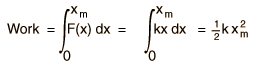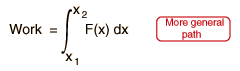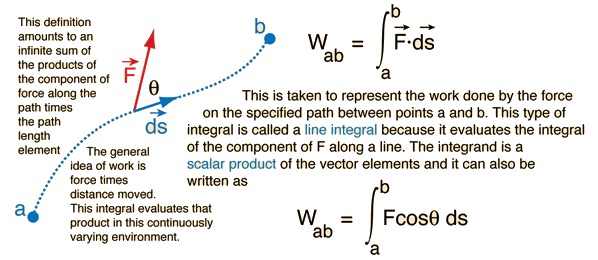# Work done by a variable forceThe basic work relationship W=Fx is a special case which applies only to constant force along a straight line. That relationship gives the area of the rectangle shown, where the force F is plotted as a function of distance. In the more general case of a force which changes with distance, the work may still be calculated as the area under the curve. For example, for the work done to stretch a spring, the area under the curve can be readily determined as the area of the triangle. The power of calculus can also be applied since the integral of the force over the distance range is equal to the area under the force curve:For any function of x, the work may be calculated as the area under the curve by performing the integralIndex

Work concepts

 HyperPhysics***** Mechanics R Nave
Go Back

# Work: General Definition

The general definition of work done by a force must take into account the fact that the force may vary in both magnitude and direction, and that the path followed may also change in direction. All these things can be taken into account by defining work as an integral.Index

Work concepts

 HyperPhysics***** Mechanics R Nave
Go Back